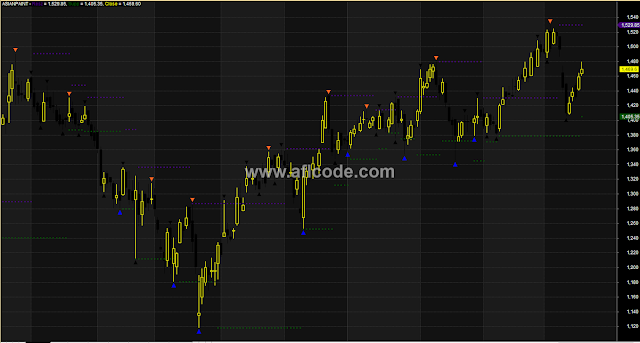### Accurate Swing Support Resistance With Buy Sell ArrowAccurate Swing Support Resistance With Buy Sell Arrow

```//www.aflcode.com
_SECTION_BEGIN("Elliot Fractals");

Up5BarFractal = Ref(H,-2) < H AND Ref(H,-1) < H AND Ref(H,1) < H AND Ref(H,2) < H;
Up6BarFractal = Ref(H,-2) < H AND Ref(H,-1) < H AND (H == Ref(H,1)) AND Ref(H,2) < H AND Ref(H,3) < H;
Down5BarFractal = Ref(L,-2) > L AND Ref(L,-1) > L AND Ref(L,1) > L AND Ref(L,2) > L;
Down6BarFractal = Ref(L,-2) > L AND Ref(L,-1) > L AND (L == Ref(L,1)) AND Ref(L,2) > L AND Ref(L,3) > L;

//TODO: More filtering: Show only troughs that are around atrough in trix(9).

PlotShapes( IIf(Down5BarFractal ,shapeSmallUpTriangle,0) ,colorBlack, 0, L,-12);
PlotShapes( IIf(Down6BarFractal ,shapeSmallUpTriangle,0) ,colorBlack, 0, L,-12);

PlotShapes( IIf(Up5BarFractal ,shapeSmallDownTriangle,0) ,colorBlack, 0, H,-12);
PlotShapes( IIf(Up6BarFractal ,shapeSmallDownTriangle,0) ,colorBlack, 0, H,-12);

Up = (Up5BarFractal OR Up6BarFractal);
Down = (Down5BarFractal OR Down6BarFractal);
//Removing false fractals:
DownSignal = Flip(Ref(Up,-1), Ref(Down,-1));
UpSignal = Flip(Ref(Down,-1), Ref(Up,-1));

LastHigh = H;
LastLow = L;

LastLowIndex = 0;
LastHighIndex = 0;
Valid = 0;
for (i=1; i < BarCount; i++)
{

LastHigh[i] = LastHigh[i-1];
LastLow[i] = LastLow[i-1];
if (Up[i])
{
Valid[i] = True;
if (DownSignal[i])
{
//Sequence of 2 Up Fractals. Validate only the higher one.
Valid[i] = H[i] >= H[LastHighIndex];
Valid[LastHighIndex] = H[LastHighIndex] > H[i];
}
LastHigh[i] = Max(H[i], H[LastHighIndex ]);
LastHighIndex = i;
}

if (Down[i])
{
Valid[i] = True;
if (UpSignal[i])
{
//Sequence of 2 Down Fractals. Validate only the lower one.
Valid[i] = L[i] <= L[LastLowIndex];
Valid[LastLowIndex] = L[LastLowIndex] < L[i];
}

LastLow[i] = Min(L[i], L[LastLowIndex]);
LastLowIndex = i;
}
}

TrixN = Trix(9);
TroughLow = Ref(TrixN, -3) > TrixN AND Ref(TrixN, -2) > TrixN AND Ref(TrixN, -1) > TrixN AND Ref(TrixN, 1) > TrixN AND Ref(TrixN, 2) > TrixN AND Ref(TrixN, 3) > TrixN;
TroughHigh = Ref(TrixN, -3) < TrixN AND Ref(TrixN, -2) < TrixN AND Ref(TrixN, -1) < TrixN AND Ref(TrixN, 1) < TrixN AND Ref(TrixN, 2) < TrixN AND Ref(TrixN, 3) < TrixN;
//TroughLow = Ref(TrixN, -2) > TrixN AND Ref(TrixN, -1) > TrixN AND Ref(TrixN, 1) > TrixN AND Ref(TrixN, 2) > TrixN;
//TroughHigh = Ref(TrixN, -2) < TrixN AND Ref(TrixN, -1) < TrixN AND Ref(TrixN, 1) < TrixN AND Ref(TrixN, 2) < TrixN;
ZeroValid = Cross(TrixN, 0) OR Cross(0, TrixN) OR Ref(Cross(TrixN, 0),1) OR Ref(Cross(0, TrixN),1);
ValidLow = TroughLow OR Ref(TroughLow, 1) OR Ref(TroughLow, 2) OR Ref(TroughLow, 3) OR Ref(TroughLow, 4);// OR Ref(TroughLow, 5));
ValidHigh = TroughHigh OR Ref(TroughHigh, 1) OR Ref(TroughHigh, 2) OR Ref(TroughHigh, 3) OR Ref(TroughHigh, 4);// OR Ref(TroughHigh, 5));

//Plot(LastHigh-10 ,"LastHigh", colorBlue, styleLine);
//Plot(LastLow-10 ,"LastLow ", colorRed, styleLine);
//Plot(Valid*5 + 10 ,"LastLow ", colorGreen, styleLine | styleThick);

//PlotShapes( IIf(Down AND Valid,shapeSmallUpTriangle,0) ,colorGreen, 0, L,-12);
//PlotShapes( IIf(Up AND Valid,shapeSmallDownTriangle,0) ,colorRed, 0, H,-12);
Maxi = Up AND (ValidHigh OR ZeroValid);
Mini = Down AND (ValidLow OR ZeroValid);
PlotShapes( IIf(Down AND (ValidLow OR ZeroValid),shapeSmallUpTriangle,0) ,colorBlue, 0, L,-12);
PlotShapes( IIf(Up AND (ValidHigh OR ZeroValid),shapeSmallDownTriangle,0) ,colorOrange, 0, H,-12);
//Plot(UpSignal*3+5,"UpSignal", colorBlue, styleLine| styleThick);
//Plot(DownSignal*3 ,"DownSignal", colorRed, styleLine| styleThick);

/*
LastMaxi = 0;
LastMini = 0;
ElliotLines = 0;
State = 0;
for (i=1; i < BarCount; i++)
{
State[i] = State[i-1];
if (Maxi[i])
{
State[i] = 1;//down
}

if (Mini[i])
{
State[i] = 2;
}

}

PlotShapes(IIf(State > 0, shapeSmallCircle, 0), IIf(State == 1, colorRed, colorBlue), 0, IIf(State == 1, H, L), -5);
*/
//Line = LineArray( x0, y0, x1, y1, 1 );
//Plot( Line, "Trend line", colorBlue );

/*
Wave B
Usually 50% of Wave A
Should not exceed 75% of Wave A
Wave C
either 1 x Wave A
or 1.62 x Wave A
or 2.62 x Wave A
*/
function CorrectiveRatios(StartPrice, A, B, C, RatioDelta, Delta)
{

ALength = abs(startPrice - A); BLength = abs(A-B);
CLength = abs(B-C);

Ratio1 = BLength / CLength ;
Cond1 = Ration1 >= 0.5 - RatioDelta AND ratio1 <= 0.75 + RatioDelta;
Cond2 = abs(Clength - ALength) < Delta OR abs(Clength - 1.62 * ALength) < Delta OR abs(CLength - 2.62 * ALength) < Delta;

return Cond1 AND Cond2;
}

function ImpulseRules(StartPrice, One, Two, Three, Four, Five)
{
//Wave 2 should be beneath wave 1 start:
Cond1 = Two > StartPrice AND Two < One;
//Wave 4 - the same:
Cond2 = Four > Two AND Four < Three;
//Wave 5 should be <= wave 3
Cond3 = abs(Three-Two) >= abs(Five - Four);
//Wave 1 should be smaller than wave five, making wave 3 the biggest:
Cond4 = abs(StartPrice - One) < abs(Five - Four);
return Cond1 AND Cond2 AND Cond3 AND Cond4;
}
_SECTION_END();

_SECTION_BEGIN("Support and Resistance");

LB= Param("Look Back Periods",10,1,30,1);
R=ValueWhen(Cross(MA(C,LB),C),HHV(H,LB),1);
S=ValueWhen(Cross(C,MA(C,LB)),LLV(L,LB),1);
Color=IIf(O>C,colorBlack,colorYellow);
Plot (R,"Resz",22,8+16);
Plot (s,"Supp",19,8+16);
Plot (C,"Close",color,64,32);
GraphXSpace=4;
_SECTION_END();
```

Previous
Next Post »